# Translating parabolas

If you translate (slide) a parabola there are four elements you need to remember:

y=x^2

1.  y=x^2+1          Goes up  uarr         (Shifts the parabola up)

2.  y=x^2-1          Goes down  darr    (Shifts the parabola down)

3.  y=(x+1)^2       Goes left  larr      (Shifts the parabola to the left)

4.  y=(x-1)^2       Goes right  rarr    (Shifts the parabola to the right)

How do you remember translating parabolas:

Draw a diagonal line as in a graph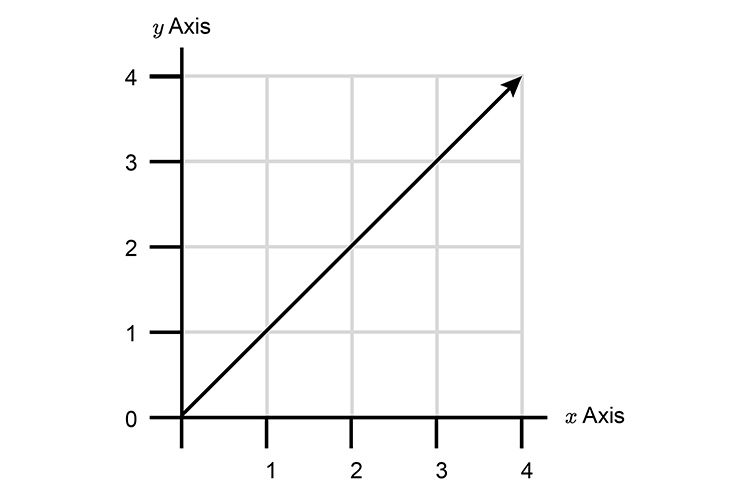Then write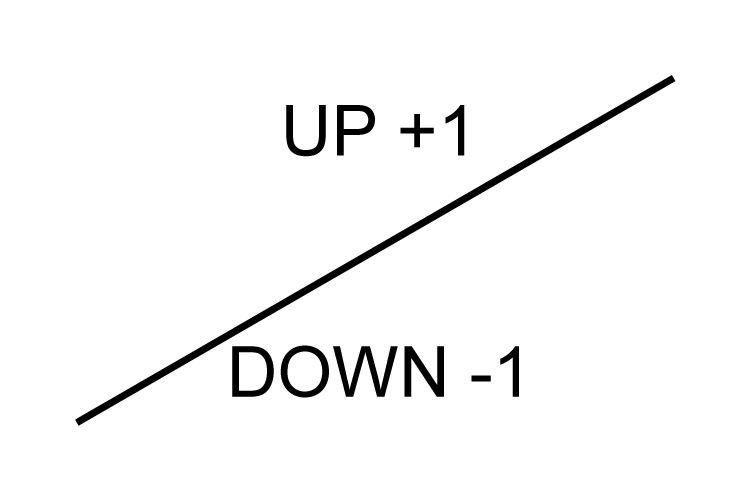Then write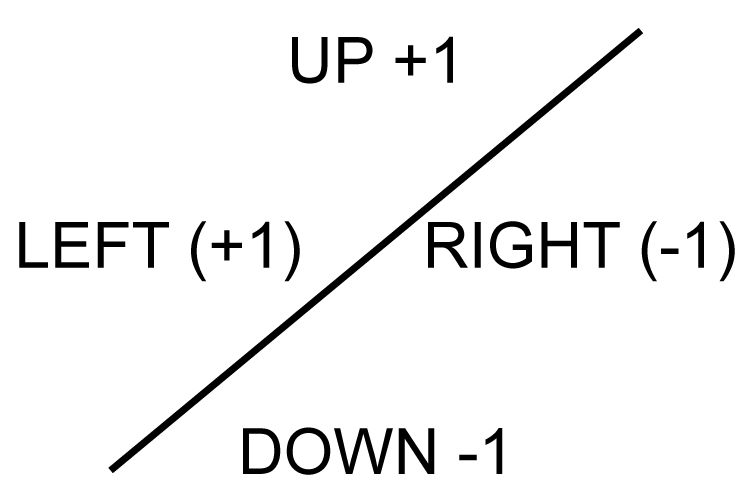This should remind you.

 UP +1 y=x^2+1 Down -1 y=x^2-1 Right (-1) y=(x-1)^2 Left (+1) y=(x+1)^2

The results would be as the following examples:

Example 1

y=x^2+1                or  Up +1

And we can prove this or further help to remember this by plotting the graph out as follows:

 x=3 y=3^2+1 =9+1 =10 x=2 y=2^2+1 =4+1 =5 x=1 y=1^2+1 =1+1 =2 x=0 y=0^2+1 =0+1 =1 x=-1 y=(-1)^2+1 =1+1 =2 x=-2 y=(-2)^2+1 =4+1 =5 x=-3 y=(-3)^2+1 =9+1 =10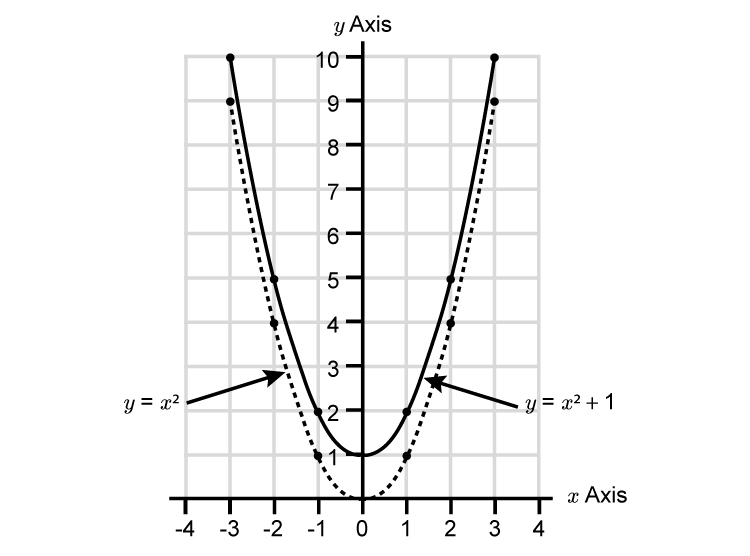The parabola has been translated by ((0),(1))

UP +1\ \ uarr

Example 2

y=x^2-1                   or  Down -1

Again we can prove this or further help to remember this by plotting the graph as follows:

 x=3 y=3^2-1 =9-1 =8 x=2 y=2^2-1 =4-1 =3 x=1 y=1^2-1 =1-1 =0 x=0 y=0^2-1 =0-1 =-1 x=-1 y=(-1)^2-1 =1-1 =0 x=-2 y=(-2)^2-1 =4-1 =3 x=-3 y=(-3)^2-1 =9-1 =8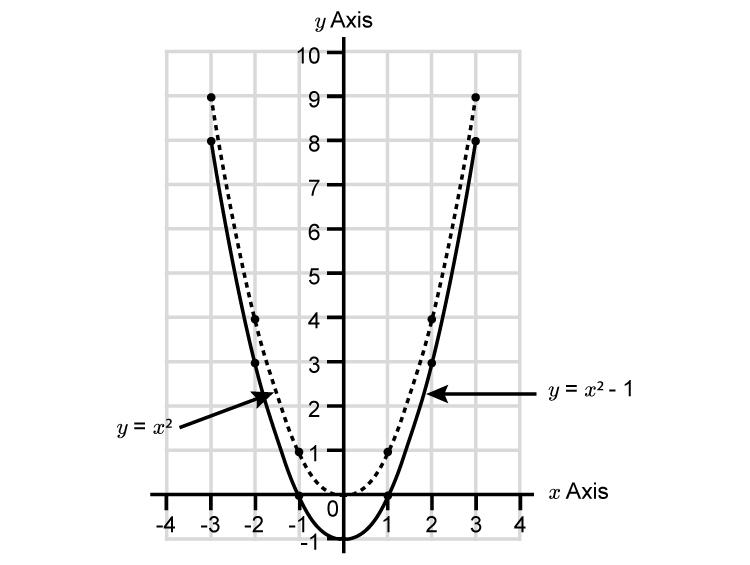The parabola has been translated by ((0),(1))

Down -1\ \ darr

Example 3

y=(x+1)^2                     or  Left larr

NOTE:

See how we added brackets around the x  value and added +1

We can prove this or further help remember this by plotting the graph as follows:

 x=3 y=(3+1)^2 =4^2 =16 x=2 y=(2+1)^2 =3^2 =9 x=1 y=(1+1)^2 =2^2 =4 x=0 y=(0+1)^2 =1^2 =1 x=-1 y=(-1+1)^2 =0^2 =0 x=-2 y=(-2+1)^2 =(-1)^2 =1 x=-3 y=(-3+1)^2 =(-2)^2 =4 x=-4 y=(-4+1)^2 =(-3)^2 =9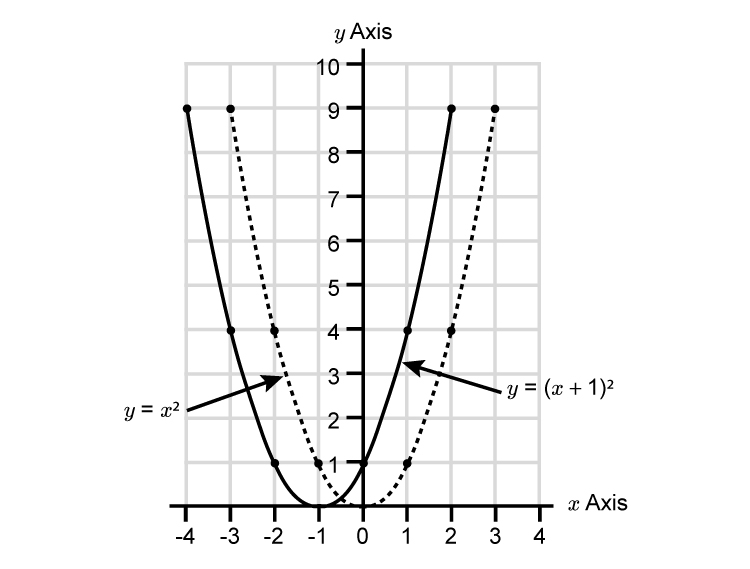The parabola has been translated by ((-1),(0))

Left -1\ \ larr

Example 4

y=(x-1)^2                    or  Right rarr

NOTE:

See how we added brackets around the x  value and subtracted 1

We can prove this or further help remember this by plotting the graph as follows:

 x=4 y=(4-1)^2 =3^2 =9 x=3 y=(3-1)^2 =2^2 =4 x=2 y=(2-1)^2 =1^2 =1 x=1 y=(1-1)^2 =0^2 =0 x=0 y=(0-1)^2 =(-1)^2 =1 x=-1 y=(-1-1)^2 =(-2)^2 =4 x=-2 y=(-2-1)^2 =(-3)^2 =9 x=-3 y=(-3-1)^2 =(-4)^2 =16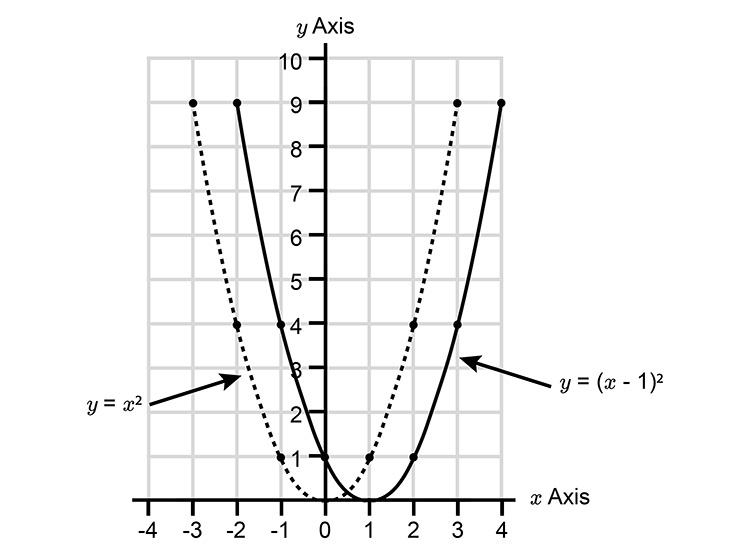The parabola has been translated by ((1),(0))

Right +1\ \ rarr